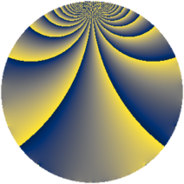# Properties

 Label 819.2.nLevel $819$ Weight $2$ Character orbit 819.n Rep. character $\chi_{819}(100,\cdot)$ Character field $\Q(\zeta_{3})$ Dimension $90$ Newform subspaces $7$ Sturm bound $224$ Trace bound $11$

# Related objects

## Defining parameters

 Level: $$N$$ $$=$$ $$819 = 3^{2} \cdot 7 \cdot 13$$ Weight: $$k$$ $$=$$ $$2$$ Character orbit: $$[\chi]$$ $$=$$ 819.n (of order $$3$$ and degree $$2$$) Character conductor: $$\operatorname{cond}(\chi)$$ $$=$$ $$91$$ Character field: $$\Q(\zeta_{3})$$ Newform subspaces: $$7$$ Sturm bound: $$224$$ Trace bound: $$11$$ Distinguishing $$T_p$$: $$2$$, $$11$$

## Dimensions

The following table gives the dimensions of various subspaces of $$M_{2}(819, [\chi])$$.

Total New Old
Modular forms 240 98 142
Cusp forms 208 90 118
Eisenstein series 32 8 24

## Trace form

 $$90 q - q^{2} - 43 q^{4} + 2 q^{5} - 2 q^{7} + 12 q^{8} + O(q^{10})$$ $$90 q - q^{2} - 43 q^{4} + 2 q^{5} - 2 q^{7} + 12 q^{8} - 2 q^{10} + 6 q^{11} + 14 q^{14} - 35 q^{16} - 11 q^{17} - 4 q^{19} + 6 q^{20} - 14 q^{22} + 5 q^{23} - 39 q^{25} + 2 q^{26} - 12 q^{28} + 4 q^{29} - 9 q^{31} - 23 q^{32} - 20 q^{34} - 9 q^{35} + 7 q^{37} - 8 q^{38} - 16 q^{40} - 7 q^{41} + 12 q^{43} + 2 q^{44} + 4 q^{46} - 10 q^{47} - 26 q^{49} + 28 q^{52} - 19 q^{53} + 26 q^{55} - 31 q^{56} + 14 q^{58} + 15 q^{59} - 28 q^{61} - 18 q^{62} - 8 q^{64} + 8 q^{65} - 20 q^{67} - 59 q^{68} - 2 q^{70} - 3 q^{71} + 3 q^{73} - 7 q^{74} - 10 q^{76} - 16 q^{77} - 20 q^{79} + 32 q^{80} - 44 q^{82} - 10 q^{83} + 13 q^{85} - 20 q^{86} + 18 q^{88} - 14 q^{89} + 29 q^{91} - 50 q^{92} - 16 q^{94} + 39 q^{95} + 40 q^{97} + 33 q^{98} + O(q^{100})$$

## Decomposition of $$S_{2}^{\mathrm{new}}(819, [\chi])$$ into newform subspaces

Label Dim $A$ Field CM Traces $q$-expansion
$a_{2}$ $a_{3}$ $a_{5}$ $a_{7}$
819.2.n.a $2$ $6.540$ $$\Q(\sqrt{-3})$$ $$\Q(\sqrt{-3})$$ $$0$$ $$0$$ $$0$$ $$5$$ $$q+(2-2\zeta_{6})q^{4}+(3-\zeta_{6})q^{7}+(3-4\zeta_{6})q^{13}+\cdots$$
819.2.n.b $2$ $6.540$ $$\Q(\sqrt{-3})$$ None $$0$$ $$0$$ $$0$$ $$5$$ $$q+(2-2\zeta_{6})q^{4}+(3-\zeta_{6})q^{7}+6q^{11}+\cdots$$
819.2.n.c $2$ $6.540$ $$\Q(\sqrt{-3})$$ None $$1$$ $$0$$ $$3$$ $$-5$$ $$q+\zeta_{6}q^{2}+(1-\zeta_{6})q^{4}+(3-3\zeta_{6})q^{5}+\cdots$$
819.2.n.d $12$ $6.540$ $$\mathbb{Q}[x]/(x^{12} - \cdots)$$ None $$-2$$ $$0$$ $$-1$$ $$9$$ $$q+(\beta _{1}+\beta _{5}-\beta _{11})q^{2}+(\beta _{6}-\beta _{7})q^{4}+\cdots$$
819.2.n.e $16$ $6.540$ $$\mathbb{Q}[x]/(x^{16} + \cdots)$$ None $$0$$ $$0$$ $$0$$ $$1$$ $$q-\beta _{1}q^{2}+(-\beta _{3}+\beta _{5}-\beta _{9}+\beta _{14}+\cdots)q^{4}+\cdots$$
819.2.n.f $20$ $6.540$ $$\mathbb{Q}[x]/(x^{20} + \cdots)$$ None $$0$$ $$0$$ $$0$$ $$-9$$ $$q+(-\beta _{1}-\beta _{4})q^{2}+(-\beta _{2}-2\beta _{7}-\beta _{16}+\cdots)q^{4}+\cdots$$
819.2.n.g $36$ $6.540$ None $$0$$ $$0$$ $$0$$ $$-8$$

## Decomposition of $$S_{2}^{\mathrm{old}}(819, [\chi])$$ into lower level spaces

$$S_{2}^{\mathrm{old}}(819, [\chi]) \cong$$ $$S_{2}^{\mathrm{new}}(91, [\chi])$$$$^{\oplus 3}$$$$\oplus$$$$S_{2}^{\mathrm{new}}(273, [\chi])$$$$^{\oplus 2}$$